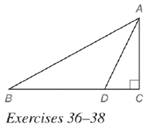Chapter 5.6, Problem 36EElementary Geometry For College St...

7th Edition
Alexander + 2 others
ISBN: 9781337614085

Solutions

Chapter
SectionElementary Geometry For College St...

7th Edition
Alexander + 2 others
ISBN: 9781337614085
Textbook Problem

In right Δ A B C , m ∠ B = 30 ∘ . Also, A B = 12 3 and A D → bisects ∠ B A C . Find B D .To determine

To find:

BD.

Explanation

Given:

In a right ΔABC with right C, AD bisects BAC and mB=30,AB=123.

Theorem used:

Angle bisector theorem:

If a ray bisects one angle of a triangle, then it divides the opposite side into segments whose lengths are proportional to the lengths of the two sides that form the bisected angle.

Calculation:

Consider a right ΔABC.

Given that mB=30,AB=123.

From the figure, sin30=ACAB

12=AC1231232=AC63=AC

Given that AD bisects BAC.

By angle bisector theorem, we get

ABAC=BDDC

ACDC=ABBD

We have AB=123,AC=63.

Still sussing out bartleby?

Check out a sample textbook solution.

See a sample solution

The Solution to Your Study Problems

Bartleby provides explanations to thousands of textbook problems written by our experts, many with advanced degrees!

Get Started

Write the number in the form a + bi. 44. ei

Single Variable Calculus: Early Transcendentals, Volume I

Evaluate the expression sin Exercises 116. (42)2

Finite Mathematics and Applied Calculus (MindTap Course List)

Name the graph of a quadratic function.

Mathematical Applications for the Management, Life, and Social Sciences

It does not exist.

Study Guide for Stewart's Multivariable Calculus, 8th